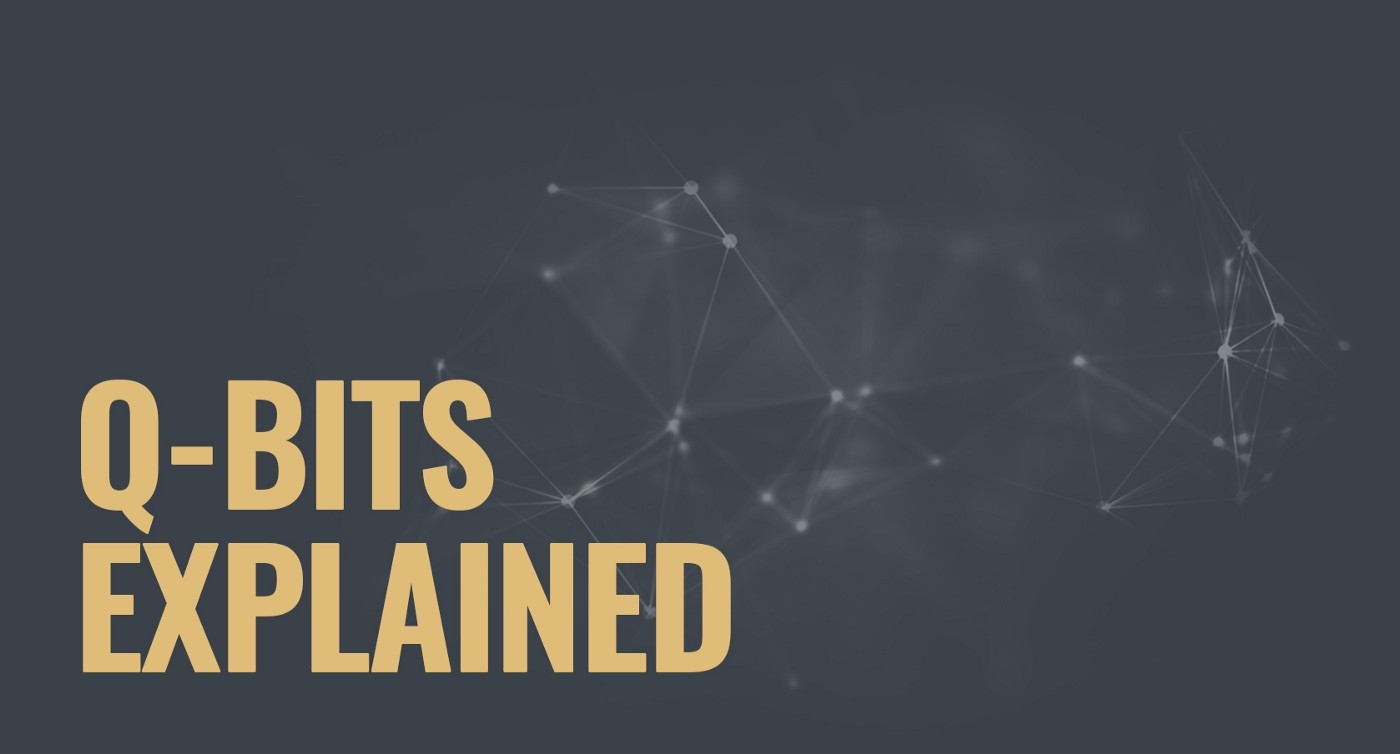# Q-Bits Explained — Part 1Quantum computer’s mentioning in the modern world steadily becoming more and more widespread in the technology field. Quantum computing is a very complexed subject, and its understanding cannot be full without going into the details with the basics — the q-bits or the quantum bits.

Basically, the qubit is a quantum bit, the counterpart in quantum computing to the binary digit or bit of classical computing. Just as a bit is the basic unit of information in a conventional computer, a qubit is the basic unit of data in a quantum computer.

However, in a quantum computer, a number of elemental particles such as electrons or photons can be used (in practice, success has also been achieved with ions), with either their charge or polarization acting as a representation of 0 and/or 1. Each of these particles is known as a qubit; the nature and behavior of these particles (as expressed in quantum theory) form the basis of quantum computing. The two most relevant aspects of quantum physics are the principles of superposition and entanglement.

Superposition

Think of a qubit as an electron in a magnetic field. The electron’s spin may be either in alignment with the area, which is known as a spin-up state or opposite to the field, which is known as a spin-down state. Changing the electron’s spin from one state to another is achieved by using a pulse of energy, such as from a laser — let’s say that we use 1 unit of laser energy. However, what if we only use half a unit of laser energy and completely isolate the particle from all external influences? According to quantum law, the particle then enters a superposition of states, in which it behaves as if it were in both states simultaneously. Each qubit utilized could take a superposition of both 0 and 1. Thus, the number of computations that a quantum computer could undertake is 2^n, where n is the number of qubits used.

A quantum computer comprised of 500 qubits would have a potential to do 2⁵⁰⁰ calculations in a single step. This is a remarkable number — 2⁵⁰⁰ is infinitely more atoms than there are in the known universe (this is accurate parallel processing — classical computers today, even so-called parallel processors, still only genuinely do one thing at a time: there are just two or more of them doing it). However, how will these particles interact with each other? They would do so via quantum entanglement.

Entanglement

Particles that have interacted at some point retain a type of connection and can be entangled with each other in pairs, in a process known as correlation. Understanding the spin state of one entangled particle — up or down — allows one to know that the spin of its mate is in the opposite direction. Even more amazing is the knowledge that, due to the phenomenon of superposition, the measured particle has no single spin direction before being measured, but is simultaneously in both a spin-up and spin-down state. The spin state of the particle being measured is decided at the time of measurement and communicated to the correlated particle, which simultaneously assumes the opposite spin direction to that of the measured particle.

This is a real phenomenon (Einstein called it “spooky action at a distance”), the mechanism of which cannot, as yet, be explained by any theory — it just must be taken as given. Quantum entanglement allows qubits that are separated by incredible distances to interact with each other instantaneously (not limited to the speed of light). No matter how high the distance between the correlated particles, they will remain entangled as long as they are isolated.

Taken together, quantum superposition and entanglement create an enormously enhanced computing power. Where a 2-bit register in an ordinary computer can store only one of four binary configurations (00, 01, 10, or 11) at any given time, a 2-qubit register in a quantum computer can store all four numbers simultaneously, because each qubit represents two values. If more qubits are added, the increased capacity is expanded exponentially.

We examine more about the fascinating quantum world within our next articles. Stay tuned.